Courses

# Revisal Problems (Past 13 Years) JEE Main (States Of Matter)

## 20 Questions MCQ Test Chemistry for JEE Advanced | Revisal Problems (Past 13 Years) JEE Main (States Of Matter)

Description
This mock test of Revisal Problems (Past 13 Years) JEE Main (States Of Matter) for JEE helps you for every JEE entrance exam. This contains 20 Multiple Choice Questions for JEE Revisal Problems (Past 13 Years) JEE Main (States Of Matter) (mcq) to study with solutions a complete question bank. The solved questions answers in this Revisal Problems (Past 13 Years) JEE Main (States Of Matter) quiz give you a good mix of easy questions and tough questions. JEE students definitely take this Revisal Problems (Past 13 Years) JEE Main (States Of Matter) exercise for a better result in the exam. You can find other Revisal Problems (Past 13 Years) JEE Main (States Of Matter) extra questions, long questions & short questions for JEE on EduRev as well by searching above.
QUESTION: 1

Solution:
QUESTION: 2

Solution:
QUESTION: 3

### The pressure of gaseous mixture of NH3 and N2 decreased from 1.5 kPa to 1.00 kPa after NH3 was absorbed from the mixture. Assuming ideal gas behaviour, mole fraction of N2 in the mixture is

Solution:
QUESTION: 4

What is the value of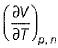for an ideal gas at 1 bar and 0°C?

Solution:
QUESTION: 5

What is the value of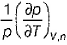at constant V ?

Solution:
QUESTION: 6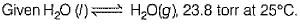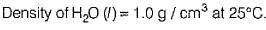Q. Thus, ratio of molar volume of water vapour to that of liquid water at 25°C is

Solution:
QUESTION: 7

A commercial gas cylinder contains 75 L of helium at 15 bar (gauge pressure). Assuming ideal gas behaviour for the isothermal expansion, how many 3.0 L balloons at a pressure of 1.1 bar can be filled by this gas in this cylinder?

Solution:
QUESTION: 8

A 1.10 L flask containing nitrogen at a pressure of 710.0 torr is connected to an evacuated flask of unknown volume. The nitrogen, which acts ideally, is allowed to expand into the combined system of both flasks isothermally. If the final pressure of the nitrogen is 5809.0 torr, volume of the evacuated flask is

Solution:
QUESTION: 9

Nitrogen dioxide (NO2) cannot be obtained in a pure form in the gas phase at exists as a mixture of NO2 and N2O4. At 298 K and 0.98 atm, the density of this gas mixture is 2.764 g L-1, Thus partial pressure of NO2 in the mixture is

Solution:
QUESTION: 10

Nitrogen forms several gaseous oxides. One of them has a density of 1.27 g L-1 at 764 mrn Hg and 150°C. Thus oxide is

Solution:
QUESTION: 11

A sample of neon gas is heated from 300 K to 390 K. Percentage increase in kinetic energy is

Solution:
QUESTION: 12

Dissolving 3.00 g of an impure sample of CaCO3 in an excess of HCI acid produced 0.656 L of CO2 (measured at 20°C and 792 mm Hg). Thus, percentage by mass of CaCO3 in the sample is

Solution:
QUESTION: 13

What will be the volume change corresponding to an increase of 0.01 mole at 25°C? The molar volume of an ideal gas under these conditions is 24.8 Lmol-1

Solution:
QUESTION: 14

Identify which of the following isotherms for a fixed amount of an ideal gas at constant temperature will not be linear?

Solution:
QUESTION: 15

The density of helium gas will be highest at

Solution:
QUESTION: 16

An empty container is filled with equal am ounts of CH4 and N2 gases. The fraction of the total pressure exerted by N2 is

Solution:
QUESTION: 17

A gas would show maximum deviations from ideal gas behaviour at

Solution:
QUESTION: 18

The values of the van der Waals’ constant (dm6 atm mol-2) for the gases are given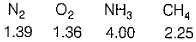Q. The gas which can be most easily liquefied is

Solution:
QUESTION: 19

Average molar m ass of air is 29.og mol-1. At the altitude of x * 103 m ,atmosphere pressure decreases from 1.00 bar to 0.50 bar. What is the value of x?

Solution:
QUESTION: 20

At the normal boiling point of water,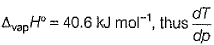for water at the boiling point, assuming that water vapour acts ideally, is

Solution: